# Periodic Solution Recurrence Relation

Probably the last post of the year- enjoy the holidays, everyone!

The problem: Periodic Solution Recurrence Relation.  This is problem AN12 in the appendix.

The description: Given a set of m ordered pairs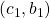through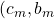with each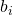>0, can we find a sequence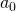though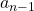of integers, such that if we build the infinite sequence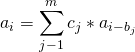is periodic: that is,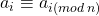for all i?

Example: Here’s a super simple example: m=2 and the pairs are (1,1) and (2,2).  This gives us the recurrence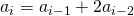.  If we start with 1,1, this gives the sequence 1,1,3,5,11,21,43,…  which is periodic mod 10 (the last digit always repeats 1,1,3,5)

Reduction: This shows up in Plaisted’s 1984 paper.  He mentions it as a corollary to his Theorem 5.1 which showed that Non-Trivial Greatest Common Divisor and Root of Modulus 1 were NP-Complete.  Similar to the Root of Modulus 1 problem, we build a polynomial from a set of clauses that has 0’s on the unit circle.  The polynomial also has a leading coefficient of 1.  This means, apparently, that the recurrence relation corresponding to the polynomial has a periodic solution if and only if the polynomial has a root on the complex unit circle, which only happens if the original 3SAT formula was satisfiable.

Difficulty: 8.